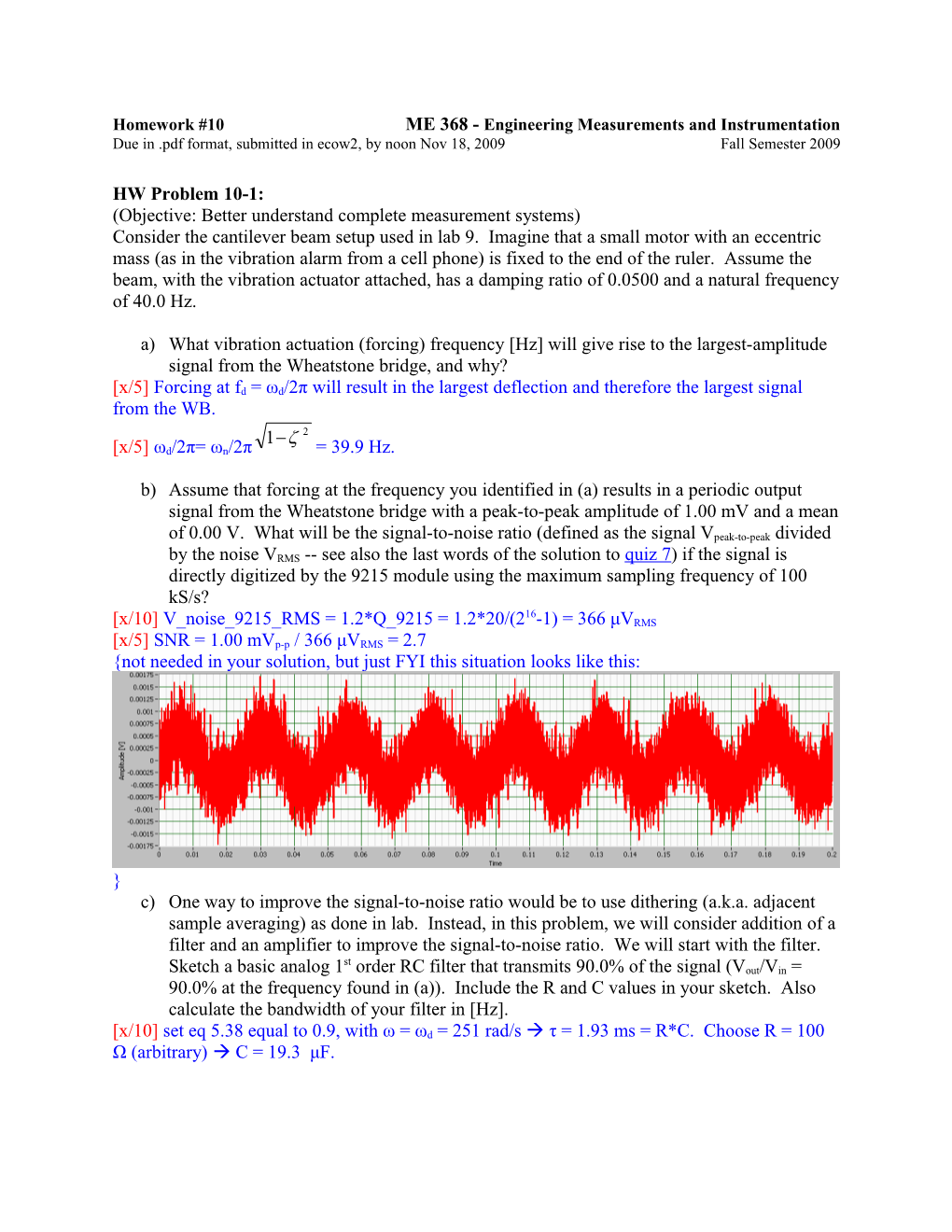# Homework #10ME 368 - Engineering Measurements and Instrumentation## Homework #10ME 368 - Engineering Measurements and Instrumentation

Duein .pdf format, submitted in ecow2, by noon Nov 18, 2009Fall Semester 2009

HW Problem 10-1:

(Objective: Better understand complete measurement systems)

Consider the cantilever beam setup used in lab 9. Imagine that a small motor with an eccentric mass (as in the vibration alarm from a cell phone) is fixed to the end of the ruler. Assume the beam, with the vibration actuator attached, has a damping ratio of 0.0500 and a natural frequency of 40.0 Hz.

a)What vibration actuation (forcing) frequency [Hz] will give rise to the largest-amplitude signal from the Wheatstone bridge, and why?

[x/5]Forcing at fd = ωd/2π will result in the largest deflection and therefore the largest signal from the WB.

[x/5]ωd/2π= ωn/2π= 39.9 Hz.

b)Assume that forcing at the frequency you identified in (a) results in a periodic output signal from the Wheatstone bridge with a peak-to-peak amplitude of 1.00 mV and a mean of 0.00 V. What will be the signal-to-noise ratio (defined as the signal Vpeak-to-peak divided by the noise VRMS -- see also the last words of the solution toquiz7) if the signal is directly digitized by the 9215 module using the maximum sampling frequency of 100 kS/s?

[x/10] V_noise_9215_RMS = 1.2*Q_9215 = 1.2*20/(216-1) = 366 μVRMS

[x/5]SNR = 1.00 mVp-p / 366 μVRMS = 2.7

{not needed in your solution, but just FYI this situation looks like this: }

c)One way to improve the signal-to-noise ratio would be to use dithering (a.k.a. adjacent sample averaging) as done in lab. Instead, in this problem, we will consider addition of a filter and an amplifier to improve the signal-to-noise ratio. We will start with the filter. Sketch a basic analog 1st order RC filter that transmits 90.0% of the signal (Vout/Vin = 90.0% at the frequency found in (a)). Include the R and C values in your sketch. Also calculate the bandwidth of your filter in [Hz].

[x/10]set eq 5.38 equal to 0.9, with ω = ωd = 251 rad/sτ = 1.93 ms = R*C. Choose R = 100 Ω (arbitrary)  C = 19.3 μF.

[x/10]sketch

[x/5] The filter bandwidth = 1/(2πτ) = 82.5 Hz

d)Assume an amplifier with a gain of 5,000 and an input noise of 0.100 [μVRMS/] is used to amplify the 1.00 mV signal described in (b). Assume the amplifier noise dominates all other noises in the system and the filter you designed in (c) sets the system bandwidth. What is the resulting signal-to-noise ratio?

[x/10]SNR = 1.00 mV * 5,000 / (100 [nVRMS/]*5,000*) = 1.10 x 103.

{not needed in your solution, but just FYI this situation looks like this: }

e)Now imagine that we won’t use the 9215 and that instead we will buy an A/D converter specifically for the task of measuring the filtered, amplified output corresponding to the 1.00 mVpeak-to-peak signal. For each row in the table below, select the best match for this task. Avoid ‘overkill’ and the associated wasted of money: choose the lowest-cost option that is sufficient (note that the options in each row are listed in order of increasing cost). Briefly justify each choice.

A/D converter property / \$ / \$\$ / \$\$\$
digitized voltage range [V] / -3 to 3 / -5 to 5 / 0 to 10
number of bits / 8 / 12 / 16
sampling frequency [Hz] / 10 / 100 / 1000
RMS noise [mV] / 1 / 0.1 / 0.01
A/D converter property / \$ / \$\$ / \$\$\$ / justification
digitized voltage range [V] / -3 to 3 / -5 to 5 / 0 to 10 / [x/10] cheapest, best fit (see plot above)
number of bits / 8 / 12 / 16 / [x/10] with 8, Q error dominates amplifier noise level
sampling frequency [Hz] / 10 / 100 / 1000 / [x/10] with 10 or 100, Nyquist frequency is lower than 82.5 Hz filter bandwidth
RMS noise [mV] / 1 / 0.1 / 0.01 / [x/10] cheapest and sufficient (amplifier noise still dominates)

{grader’s note: many students had a different interpretation of basically “select one column in the table” so Prof. Sanders suggests all graders be lenient / open-minded while grading of this table.}